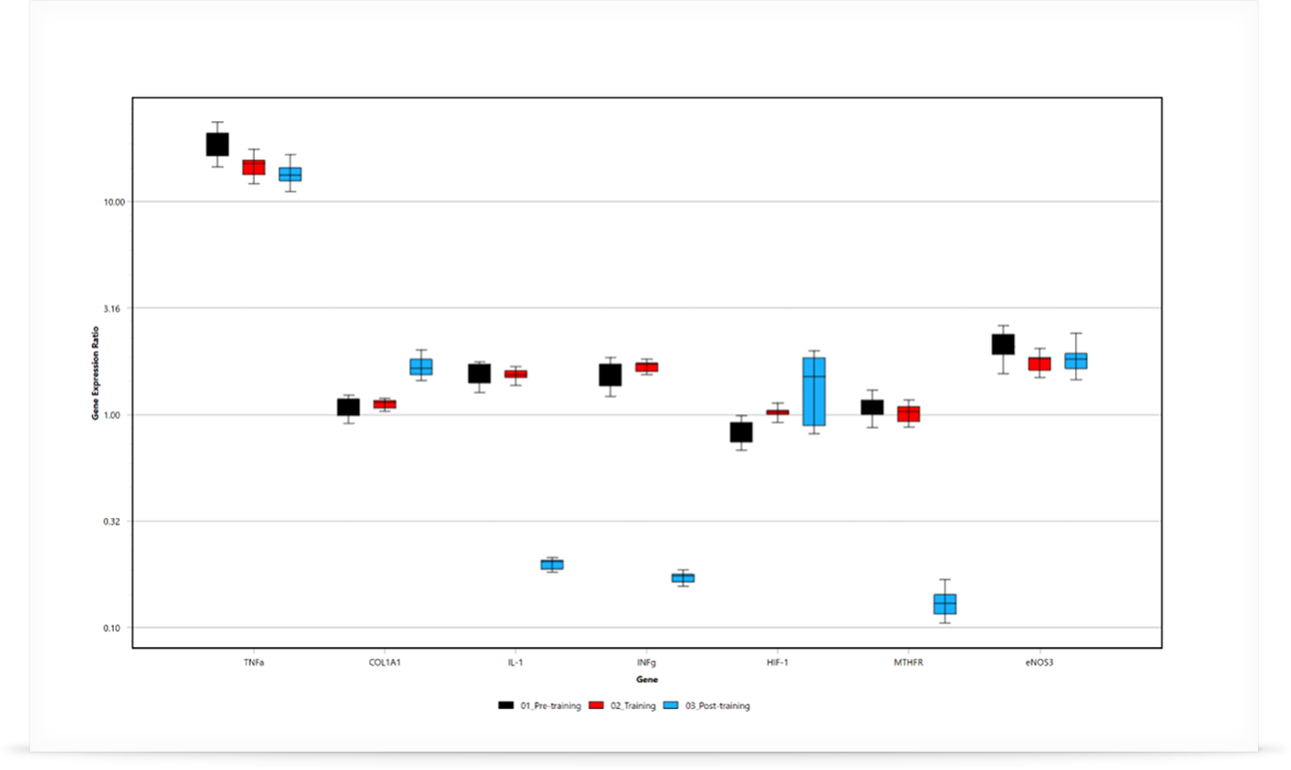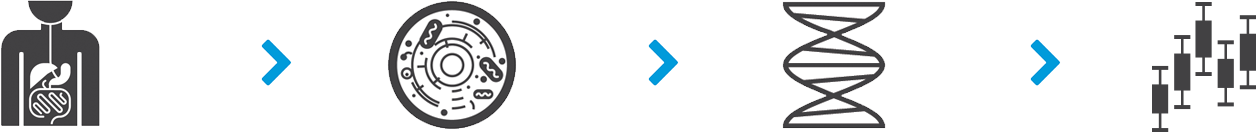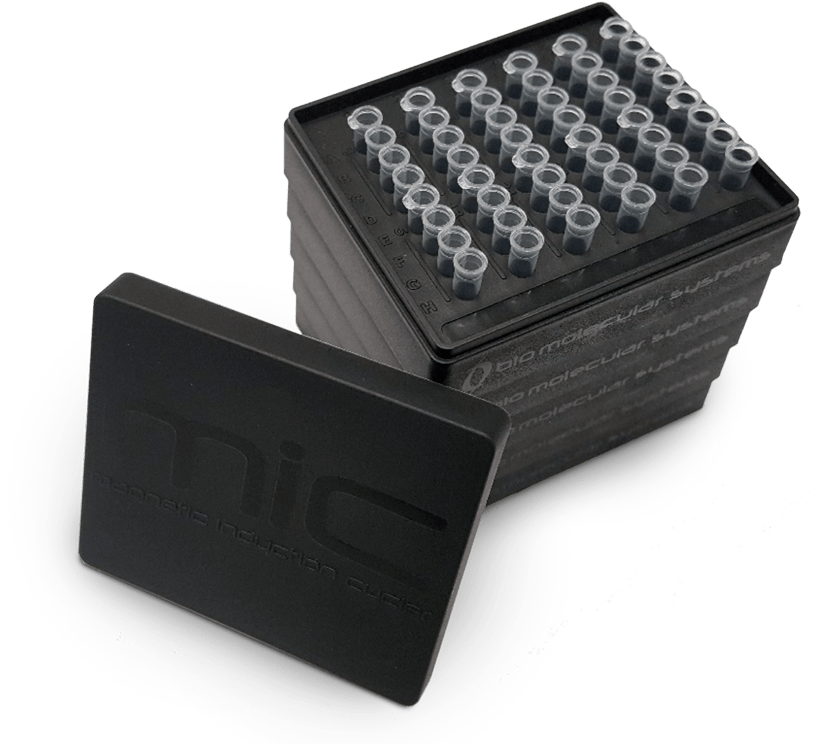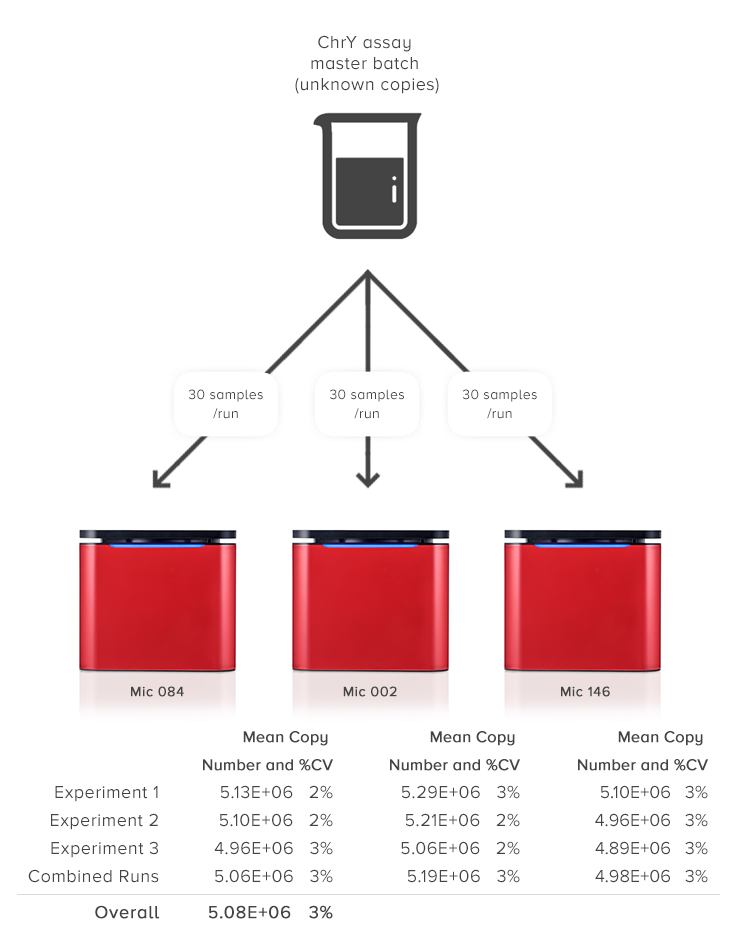# Gene Expression

#### Relative Quantifications Analysis using micPCR Software.## Gene Expression

#### The Basics

Using real-time PCR (qPCR) for gene expression analysis has been an established method for some time now. The simplest aim of gene expression analysis is to compare the amount of mRNA for specific targets between a treatment group and a control group to help better understand the normal or disease state of any biological system.

The process begins with the extraction and isolation of mRNA from a biological sample such as tissue or liquid biopsy. As the mRNA cannot be used in PCR, it must first be converted to cDNA using reverse transcription (RT). RT-qPCR can be achieved in either a one-step or two step procedure. To account for variations in starting template and RT efficiency, an endogenous housekeeping gene is also amplified. This reference gene is used to normalise for the variations, and as such it must be proven to not vary in expression profile between treatment and control groups. The geNorm method developed by Jo Vandesompole et al. in 2002 is one such method for determining reference gene stability between groups.## Determining Cq

Once the RT-qPCR is completed, the resulting real-time curves are analysed to determine the gene expression ratio between the treatment and control group. The first step is to determine the quantification cycle (Cq) for each sample of each gene being investigated. One approach is to use the LinRegPCR method developed by Jan Ruijter et al. in 2003 and published again in 2009.

The LinRegPCR algorithm utilises the region of exponential growth to calculate Cq values, while minimising the variation among the dataset, thus avoiding the potential for miscalculations of efficiency and starting target concentrations due to either over or under estimating the baseline subtraction. This is a highly accurate and reproducible method to calculate Cq values, which has the added benefit of reporting an efficiency value for each sample rather than using a standard curve. Using individual efficiencies removes the issue of random pipetting errors during dilution of the standards or the dilution of PCR inhibitors, both issues that can introduce errors in the standard curve (Ramakers et al. 2003; Ruijter et al. 2009). Other baseline correction methods and threshold setting strategies can be employed to determine Cq values as well.• Example of LinRegPCR analysis. The qPCR curves are displayed with the y-axis in log scale to better emphasise the region of exponential growth. The second derivative maximum is used as a starting point to locate a Window-of-Linearity (WoL; shaded area). A cycle threshold (red line) is automatically set within the WoL from which a Cq value can be determined for each sample. Using the gradient of the line for each sample in the WoL, the reaction efficiency is also calculated.

## Calculating Expression Ratios

Once the Cq values have been determined for the gene of interest and reference gene, the relative gene expression ratio (R.E.) can be calculated. The simplest equation for qPCR analysis describes the determination of the starting concentration using the amount of target at the quantification cycle (Cq) and efficiency of the reaction.Where N0 is the starting concentration, Nq is the concentration at the Cq, and E is the reaction efficiency.

The equation developed by Michael Paffl in 2001 was the first to ensure each individual reaction efficiency was used. This is critical because if the difference in reaction efficiency between targets is not taken into account, the final result can be significantly biased.Where E is the calculated reaction efficiency for each gene.

An alternative method – one that is still popular today – is delta-delta Ct (ΔΔCt), published by Livak and Schmittgen in 2001.
This method assumes the efficiency is 100% which is acceptable as long as it has been validated that the gene of interest and reference gene have similar efficiencies.

## Statistical Analysis

The final part of the analysis is to determine whether there is statistical meaning to the gene expression ratio between the groups analysed. A robust method of doing this is the Relative Expression Software Tool (REST), first published by Michael Paffl et al. in 2002 and later improved in 2009. This method is considered to have strong statistical foundations as it assumes that the expression ratios are not normally distributed.  Most data sets are not normally distributed.

REST analysis utilises non-parametric bootstrapping techniques to calculate the appropriate errors and probability (P) value for the comparison between groups.  The distribution of the randomly generated observations is used to determine significance.  If the mean calculated gene expression ratio falls outside of more than 95% of the observations, then it can’t be random and it is considered statistically significant (P ≤ 0.05).

In the case that the data has been shown to be normally distributed, an alternative method using a student t-test can be also used. This method is typically applied when using the ΔΔCt method, however care must be taken to confirm the data distribution first. REST will work for all data sets.

## Multi-experiment Analysis

It is now very common to analyse multiple experiments in one analysis.

There are a number of methods in use for normalising data sets across multiple experiments

1. Normalisation between experiments: This method adds variation due to the control having a margin of error.
2. Standardisation: This method can bias the data if the data set is incomplete.
3. Factor Correction: Uses unbiased measures within an experiment to ensure a smaller residual error.

Based on the published method of Jan Ruijter et al. in 2006 Factor-qPCR can be applied to all of the data sets to ensure the most accurate comparison between experiments. This correction allows for the detection of smaller differences between the control and treatment groups, without the need for an internal reference calibrator.• Combine multiple Mic runs into one gene expression analysis.

*Due mid-2019

## Results

#### Clear and Concise

The results are graphically displayed with various options available such as plotting genes or groups, linear or log scale, and colour selection. Each graph can easily be exported at the click of a button. Remove or add groups or targets with ease using the analysis parameters available.

The table of results is simple and informative with all the statistics shown in one easy to read format. Export the data to Microsoft® Excel® or Clipboard® for hassle-free publication.

## Projects

#### Multi-Run Analysis

Easily analyse multiple experiments in a single Project. Expect assured results due to Mic’s amazing reproducibility between runs and instruments (CV < 5%). This type of reproducibility is within the range of the expected Poisson distribution, especially for low copy numbers that can be as high as 6%.  For some block based cyclers you can expect upwards of 10%.

Combine over 400 samples in one analysis without the need to batch into a 384 well plate.• Unbeatable reproducibility means combining runs into a single analysis is a non-issue. The coefficient of variability between experiments and instruments is no greater than 5%.